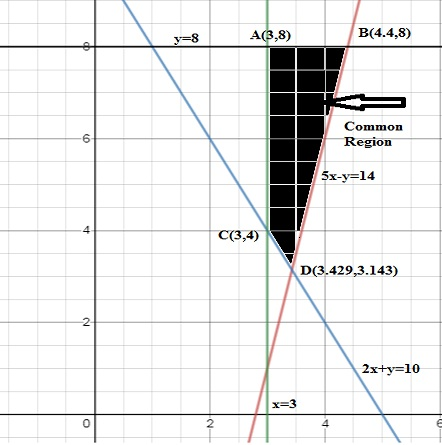# Solve the linear programming problem. Maximize z = 6x + 2y subject to 5x - y \leq14 2x + y...

## Question:

Solve the linear programming problem. Maximize

z = 6x + 2y

subject to

{eq}5x - y \leq14 {/eq}

{eq}2x + y \geq 10 {/eq}

{eq}x \geq 3 {/eq}

{eq}y\leq 8 {/eq}

## Linear Programming:

The above question concerns the topic of linear programming. This question is solved by the graphical method. In the graphical method, first, we determine the common region, in which all the constraints satisfy. After that, we find the function's maximum or minimum value at the boundary points. The point which gives the maximum or minimum value is the solution f that problem.

We have to solve the linear programming problem

{eq}\begin{align} \text{Max } z &= 6x + 2y---(1) \end{align} {/eq}

subject to the given constraints

{eq}\begin{align} 5x - y &\leq14---(2)\\ 2x + y &\geq 10---(3)\\ x &\geq 3---(4)\\ y &\leq 8---(5) \end{align} {/eq}

First we draw all the lines and find the common region. Following figure shows the common region.To find the boundary point, we solve the above equations

Solving equation (4) and (5), we have

{eq}x=3 \text{ and } y=8\\ \text{ so point is } A(3,8) {/eq}

Solving equation (2) and (5), we have

{eq}\displaystyle y=8 \text{ and } x=\frac{14+y}{5}= 4.4 \\ \text{ so point is } B(4.4,8) {/eq}

Solving equation (3) and (4), we have

{eq}\displaystyle x=3 \text{ and } y=10-2x= 4 \\ \text{ so point is } C(3,4) {/eq}

Adding equation (2) and (3), we have

{eq}\displaystyle 7x=24 \text{ and } x= 3.429 \\ \text{and } y=10-2x=3.143 \text{ so point is } D(3.429,3.143) {/eq}

Now finding the value of Z on boundary points, we have

Z=6x+2y
At point   A(3,8) Z=6(3)+2(8) = 34
At point   A(4.4,8) Z=6(4.4)+2(8) = 42.4 (max)
At point   A(3,4) Z=6(3)+2(4) = 26
At point   A(3.429,3.143) Z=6(3.429)+2(3.143) = 26.86

From above table, it is clear that the maximum value of Z is at point {eq}B(4.4,8) {/eq} and the maximum value is {eq}\text{Max. Z}=42.4 {/eq}